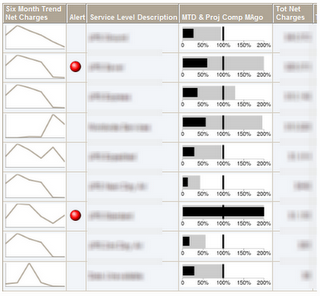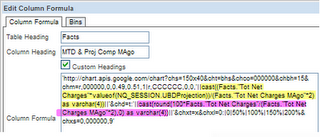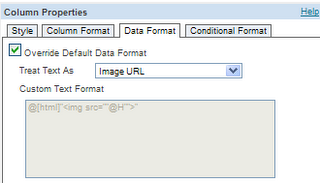# KPI Partners Blog

### by Kurt Wolff

Stephen Few designed the Bullet Graph as a way to display measurements vs. goals or other benchmarks. The screen shot below shows bullet charts in the column “MTD & Proj Comp MAgo” (Month to Date and Projected Expense Compared to Month Ago).The black horizontal bars show the current month to date expense as a percentage of the previous month’s expense amount. The gray horizontal bars show the projected expenses for the current month, assuming linearity. The black vertical bar, which is set at 100%, represents the level of expense in the previous month.

These bullet charts are done using the google charting api.

To generate these bullet charts OBIEE needs to generate a url that contains the right parameters. Other web sites have done a good job documenting the parameters of the url. For example, see http://dealerdiagnostics.com/blog/2008/05/create-bullet-graphs-with-google-charts-in-7-easy-steps/ .

In this case, the url includes several parameters, each separated by an ampersand, and breaks down like this:

chs=150x40 size of the chart

&cht=bhs type of chart, horizontal bar

&chco=000000 color of the bar = black

&chbh=15 bar width

&chm=r,000000,0,0.49,0.51,1 vertical line (thin bar) from 49% to 50%

|r,CCCCCC,0,0,'||cast((Facts."Tot Net Charges"*valueof(NQ_SESSION.UBDProjection))/(Facts."Tot Net Charges MAgo"*2) as varchar(4))||' gray horizontal bar (projected amount)

&chd=t:'||cast(round(100*Facts."Tot Net Charges"/(Facts."Tot Net Charges MAgo"*2),0) as varchar(4))||' data as a percent of 2*MAgo

&chxt=x says to label the x axis

&chxl=0:|0|50%|100%|150%|200% labels for the x axis

&chxs=0,000000,9' specifies the first label (0), color, and size

The whole URL looks like this:The only two parts of this that reference data from the query are the parts highlighted in yellow and purple.

The yellow formula draws the gray bar (color = #CCCCCC) that starts at 0 and ends at a point represented by the fraction being computed by the yellow formula. The formula multiplies the total net charges for the current month by the Projection session variable (days in month/current day of month), then divides that by 2 times the total net charges last month. Since this is going to be part of a url and has to be concatenated with other text, the cast as varchar is needed.

The purple formula is the length of the black bar. Again, that is expressed as a fraction (actually here, a percentage) of 2 times the expenses of the previous month. The cast as varchar is needed here, too.

It’s a little inconsistent in that the black horizontal bar is represented by a number (0 to 100), while the gray bar starts at 0 and extends to a number that has a value between 0 and 1 (projected expense/(2 * MAgo)). The black “vertical line” is a bar that starts at 0.49 and ends at 0.51.

The final step is to set the column’s data format to Image URL.Tags: Kurt Wolff, Oracle BI, Blog